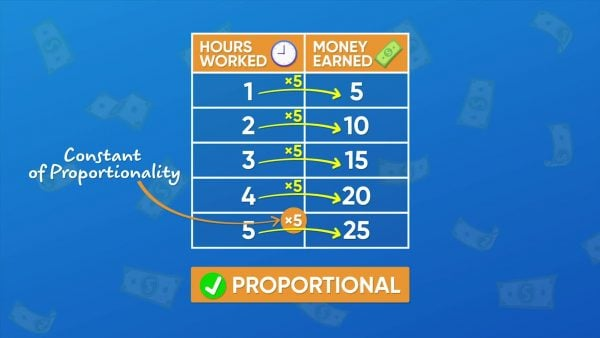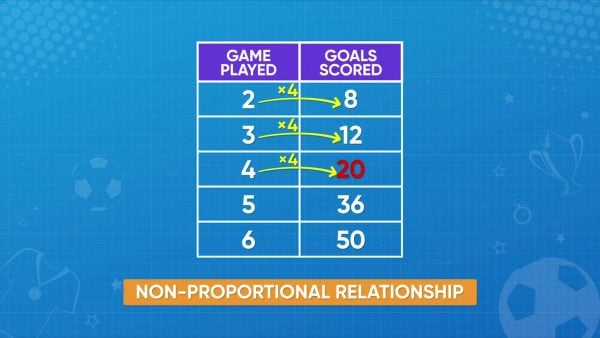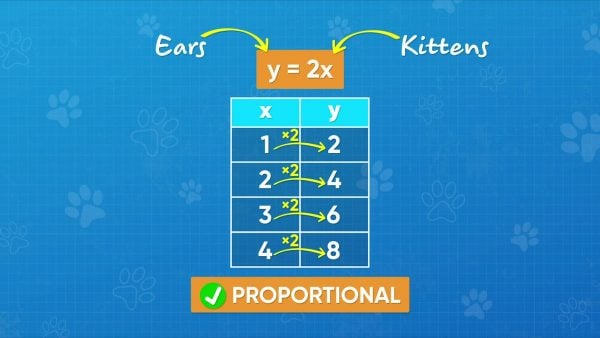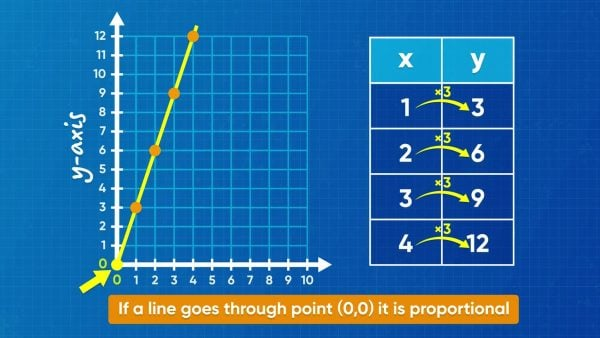Proportional Relationships | Math for kids Grade 6, 7, & 8
1%
It was processed successfully!WHAT ARE PROPORTIONAL RELATIONSHIPS?

A relationship is proportional if each pair of data values are related in the same way, by multiplying by a factor. You can recognize a proportional relationship by looking at data, an equation, or a graph.

To better understand proportional relationships…

WHAT ARE PROPORTIONAL RELATIONSHIPS?. A relationship is proportional if each pair of data values are related in the same way, by multiplying by a factor. You can recognize a proportional relationship by looking at data, an equation, or a graph. To better understand proportional relationships…

## LET’S BREAK IT DOWN!

### Make muffins using a proportional relationship.To make 1 dozen muffins, you need 2 eggs. If you want to make 2 dozen muffins, you need 4 eggs. To make 3 dozen muffins, you need 6 eggs. 1 times 2 is 2, 2 times 2 is 4, 3 times 2 is 6. The number of eggs is always 2 times the number of batches of muffins. This is called a proportional relationship. Now you try: How can you tell if the following data represents a proportional relationship? 1:3, 2:6, 3:9, 4:12

Make muffins using a proportional relationship. To make 1 dozen muffins, you need 2 eggs. If you want to make 2 dozen muffins, you need 4 eggs. To make 3 dozen muffins, you need 6 eggs. 1 times 2 is 2, 2 times 2 is 4, 3 times 2 is 6. The number of eggs is always 2 times the number of batches of muffins. This is called a proportional relationship. Now you try: How can you tell if the following data represents a proportional relationship? 1:3, 2:6, 3:9, 4:12

### The constant of proportionality is the multiplication factor.Adesina works different numbers of hours at a skating rink. The amount of money she makes for each number of hours she works is represented by this relationship: 1:\$5, 2:\$10, 3:\$15, 4:\$20, 5:\$25. Is the relationship proportional? 1 times 5 is 5, 2 times 5 is 10, and so on. The number of dollars earned is always 5 times the number of hours worked. The relationship is proportional. The number that you always multiply by is called the constant of proportionality. The constant of proportionality of this relationship is 5. Now you try: What is the constant of proportionality for the following set of ratios? 2:14, 3:21, 4:28, 5:35

The constant of proportionality is the multiplication factor. Adesina works different numbers of hours at a skating rink. The amount of money she makes for each number of hours she works is represented by this relationship: 1:\$5, 2:\$10, 3:\$15, 4:\$20, 5:\$25. Is the relationship proportional? 1 times 5 is 5, 2 times 5 is 10, and so on. The number of dollars earned is always 5 times the number of hours worked. The relationship is proportional. The number that you always multiply by is called the constant of proportionality. The constant of proportionality of this relationship is 5. Now you try: What is the constant of proportionality for the following set of ratios? 2:14, 3:21, 4:28, 5:35

### You can identify if a relationship is proportional.The following data shows the number of soccer games you played related to the number of goals you scored: 2:8, 3:12, 4:20, 5:36, 6:50. 2 times 4 is 8, and 3 times 4 is 12. But 4 times 4 is 16, not 20. And 5 times 4 is 20, not 36. You can't always multiply the number of games played by the same number to get the number of goals scored. This is a non-proportional relationship. Now you try: Identify if the following data represents a proportional relationship: 3:9, 5:15, 6:24, 8:32

You can identify if a relationship is proportional. The following data shows the number of soccer games you played related to the number of goals you scored: 2:8, 3:12, 4:20, 5:36, 6:50. 2 times 4 is 8, and 3 times 4 is 12. But 4 times 4 is 16, not 20. And 5 times 4 is 20, not 36. You can't always multiply the number of games played by the same number to get the number of goals scored. This is a non-proportional relationship. Now you try: Identify if the following data represents a proportional relationship: 3:9, 5:15, 6:24, 8:32

### You can describe proportional relationships using equations.Every kitten has 2 ears. You can write an equation to show how the number of kittens is related to the number of ears. Let x be the number of kittens and y be the number of ears. So, y=2x, since the number of ears is always twice the number of kittens. You can plug in any number of kittens for x to find the number of ears, y. For example, 5 kittens have y = 2 × 5 = 10 ears. Now you try: Write an equation to describe the relationship between the number of dogs and the number of legs in all.

You can describe proportional relationships using equations. Every kitten has 2 ears. You can write an equation to show how the number of kittens is related to the number of ears. Let x be the number of kittens and y be the number of ears. So, y=2x, since the number of ears is always twice the number of kittens. You can plug in any number of kittens for x to find the number of ears, y. For example, 5 kittens have y = 2 × 5 = 10 ears. Now you try: Write an equation to describe the relationship between the number of dogs and the number of legs in all.

### You can recognize proportional relationships in equations and graphs.Equations that represent proportional relationships are always in the form y=kx, where k is the constant of proportionality. That means that the relationship between x and y is always multiplicative, with nothing added or subtracted. Unlike y=2x, y=2x+4 and y=2x-5 do not represent proportional relationships. 4 is added or 5 is subtracted from the product. The graph of a proportional relationship is always a straight line that passes through the origin, (0, 0). Now you try: Identify which equation(s) represent proportional relationships: a) y=7.7x b) y=3x+5 c) y=13x

You can recognize proportional relationships in equations and graphs. Equations that represent proportional relationships are always in the form y=kx, where k is the constant of proportionality. That means that the relationship between x and y is always multiplicative, with nothing added or subtracted. Unlike y=2x, y=2x+4 and y=2x-5 do not represent proportional relationships. 4 is added or 5 is subtracted from the product. The graph of a proportional relationship is always a straight line that passes through the origin, (0, 0). Now you try: Identify which equation(s) represent proportional relationships: a) y=7.7x b) y=3x+5 c) y=13x

## PROPORTIONAL RELATIONSHIP VOCABULARY

Proportional relationship
When two variables are always related in the same way through multiplication.
Constant of proportionality
The number that you always multiply by to define a proportional relationship.
Non-proportional relationship
When variables are not always related in the same way.
Equations
Two expressions that have the same value and are separated by an equal sign.
Coordinate Plane
A graph that uses two variables to describe the location of a point.
X-axis
The horizontal line on a graph.
The vertical line on a graph.

## PROPORTIONAL RELATIONSHIP DISCUSSION QUESTIONS

### Each person gets 3 scoops of ice cream. Is the relationship between the number of people and the number of scoops proportional?

It is proportional. 1 person means 3 scoops, 2 people means 6 scoops, 3 people means 9 scoops. I always multiply the number of people by 3 to get the number of scoops.

### Is this relationship proportional? 3:9, 5:15, 6:18, 7:14, 8:16

No, it is non-proportional. Some of the number pairs are related by multiplying by 3, but some of them are related by multiplying by 2.

### What is the constant of proportionality for this set of number pairs? 1:3.5, 2:7, 3:10.5, 4:14

The constant of proportionality is 3.5. I can always find the constant of proportionality by dividing the second number in a pair by the first number. For each pair, multiply the first number by 3.5 to get the second number.

### Is y=2x+1 a proportional relation?

No. All proportional relationships are represented by equations that relate variables only through multiplication and are in the form y=kx, where k is any number. Since 1 is added in this equation, it is not proportional.

### Graph the points (1, 0.5), (2, 1), (4, 2), and (6, 3). Does the graph represent a proportional relationship?

Yes, if I connect the points and extend a line through them, the line goes through the origin.
X

## Success

We’ve sent you an email with instructions how to reset your password.
Ok